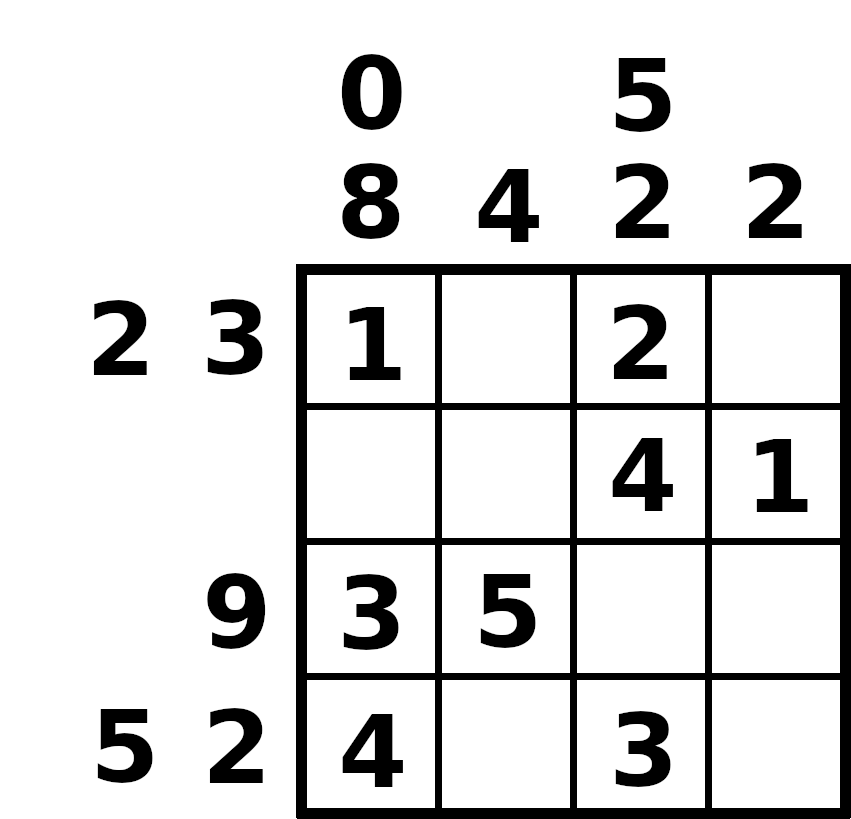#FliegendeWurst's corner of the WWW

## Solving the Logelei off-by-one puzzle using Z3

2022-11-30

In this blog post, I'll describe how to solve a logic puzzle using Z3, an automated SMT solver.

### The puzzle

You are given a rectangular grid where each cell may either contain a number (between 1 and 5) or be empty. Each number may only be used once in each row and column. In each row, the empty cells split the numbered cells into segments. The sums of these segments are indicated to the left of the grid. The same applies to the columns of the grid (sums are indicated above the grid). But there's a twist: due to a transmission error, all of the sums are off by one! The (already solved) 4-by-4 grid below illustrates these constraints.I originally found this puzzle in an edition of the ZEIT, a weekly German newspaper. See the previous link for a more difficult puzzle. It is solvable without computer assistance, but quite tricky!

### Prerequisites

To follow along, you'll first need to install Python. Then, you need to install the Z3 Python bindings. The precise installation steps vary by operating system, check the official documentation for more details.

### Defining the board

In the following, I will describe the code required to explain the puzzle to Z3. First, we need to import the z3 Python module. To avoid prefixing every constructor with z3., we import all symbols into the local namespace. We also import the math module (we will need it later). We also create a new Solver object for later use.

``````from z3 import *
import math

s = Solver()
``````

Problems are specified by creating a bunch of variables (booleans, integers, ...) and constraints on these variables. Z3 will then produce a model that assigns a value to each variable if the constraints are satisfiable. Since we are interested in the values of the grid cells, we create an integer variable for each cell. FreshInt returns a new integer variable that isn't identical to any other previously created variable.

``````# n*n grid
n = 6

grid = [[FreshInt() for x in range(n)] for y in range(n)]
``````

### Specifying the constraints

Empty grid cells will be indicated by a value of zero. All other cells need to be filled with a number between one and five. To implement this constraint, we iterate over each row and cell of the grid and add the constraint (integer value must be at least 0 and at most 5) to the solver.

``````for row in grid:
for cell in row:
``````

For each row/column, the numbers used must be unique. This is modeled by pairwise inequality of the variables making up that row or column. Of course, a value of zero (= empty grid cell) may appear more than once.

We define a function distinct_if_nonzero that, given a list of variables, adds constraints to ensure that any two variables are not equal if both of them are nonzero. This is done by supplying three parameters to the If function: the condition, the constraint if the condition is true, the constraint if the condition is false.

``````def distinct_if_nonzero(x):
for i in range(len(x)):
for j in range(i+1, len(x)):
cell_i = x[i]
cell_j = x[j]
s.add(If(And(cell_i != 0, cell_j != 0), cell_i != cell_j, True))
``````

Then we simply apply this function to each row and column of the grid.

``````for row in grid:
distinct_if_nonzero(row)

for column in [[grid[y][x] for y in range(n)] for x in range(n)]:
distinct_if_nonzero(column)
``````

The sums indicated next to the grid are represented by integer variables. It is quite obvious that the maximum number of segments possible in each row is achieved if every second cell is filled with a number. As such, we only need to keep track of that many segment sums.

``````num_sums = math.ceil(n / 2)
sums_h = [[FreshInt() for i in range(num_sums)] for y in range(n)]
sums_v = [[FreshInt() for i in range(num_sums)] for x in range(n)]
``````

Connecting these sums to the cell variables is done by considering each binary pattern p of numbered / not numbered cells.

``````# iterate over each row of the grid
for y in range(n):
# iterate over each binary pattern
for p in range(2**n):
# match condition of this pattern
mc = True
# will contain a list of variables for each segment
segments = []
start_next_segment = True
# iterate over each grid cell
for x in range(n):
# check that binary digit in the pattern
if (p >> x) & 1 == 1:
mc = And(mc, grid[y][x] != 0)
if start_next_segment:
segments.append([grid[y][x]])
start_next_segment = False
else:
segments[-1].append(grid[y][x])
else:
mc = And(mc, grid[y][x] == 0)
start_next_segment = True
# specify sums value of each segment
for k in range(num_sums):
if k < len(segments):
off_by_one = Or(
sums_h[y][k] == sum(segments[k]) + 1,
sums_h[y][k] == sum(segments[k]) - 1
)
else:
``````

The same is done for the columns of the grid. For brevity, that code is omitted from this post. The full source code is linked at the end of the article.

### Finding and displaying a solution

We still need to encode the off-by-one sums given in the puzzle as constraints. This is fairly simple:

``````def add_spec(spec_h, spec_v):
for a, b in zip(spec_h, sums_h):
for x, y in zip(a, b):
for a, b in zip(spec_v, sums_v):
for x, y in zip(a, b):
spec_h = [
[2, 13, -1],
[5, 3, 6],
[8, 7, -1],
[4, 4, 1],
[5, 5, -1],
[9, 0, -1]
]

spec_v = [
[11, -1, -1],
[4, 5, -1],
[7, 7, -1],
[2, 7, 3],
[4, 4, 5],
[10, 2, -1]
]
s.push()
``````

Note that we used s.push() to create a nested constraint context. Any constraints added after this call may be removed by calling s.pop(). We will make use of this later to generate new puzzles (with new off-by-one sums).

Now that we have specified all of our contraints, we may ask Z3 to determine the validity of our model. s.check() may return sat, unsat or unknown. sat indicates that the problem is satisfiable (there is a variable assignment that fulfills all constraints), unsat indicates that no such assignment is possible. Z3 only returns unknown if it is not able to deduce whether the problem is satisfiable or not (e.g., due to incomplete theories).

The loop below uses a simple trick to enumerate all solutions to the puzzle: after one solution is found, add a constraint that at least one grid cell must be different.

``````res = s.check()
print(res)

while res == sat:
m = s.model()
print_solution(m)
# find another solution, if possible
c = False
for row in grid:
for cell in row:
c = Or(c, cell != m[cell].as_long())
res = s.check()
print("no other solutions")
s.pop()
``````

### Generating puzzles with a unique solution

Generating new puzzles is surprisingly easy at this point. It boils down to not constraining the off-by-one sums. Z3 will still generate a model that fits all of the puzzle rules. To determine whether a newly-found puzzle has a unique solution, we first need to add the new off-by-one sums to Z3. Additionally, we mandate that at least one grid cell needs to differ. If no other solution is found, we know that the puzzle has a unique solution. Otherwise, we add a constraint that bans this particular off-by-one sums configuration (this ensures we always try new puzzles).

``````# try to find another puzzle with a unique solution
while True:
s.push()
print("new push")
print(s.check())
m = s.model()
# condition c: keep found off-by-one sums configuration
# condition c2: to ban this sums configuration
c = True
c2 = False
for l in [*sums_h, *sums_v]:
for x in l:
c = And(c, x == m[x].as_long())
c2 = Or(c2, x != m[x].as_long())
# try to find another solution
c = False
for row in grid:
for cell in row:
c = Or(c, cell != m[cell].as_long())
res = s.check()
print(res)
if res == unsat:
print("found another puzzle with unique solution")
print_solution(m, print_grid=False)
input("continue? [press enter]")
s.pop()